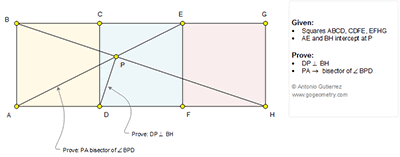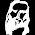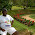## Sunday, August 2, 2015

### Geometry Problem 1144: Three Equal Squares, Diagonals, Perpendicular, 90 Degrees, Angle Bisector

Geometry Problem
Level: Mathematics Education, High School, Honors Geometry, College.

Click the figure below to see the complete problem 1144.#### 8 comments:

1.∠APB = ∠PEB + ∠PBE = arctan(1/2) + arctan(1/3) = 45°
thus A B C P D are concyclic.

Since BD is the diameter, ∠BPD = 90°.
Thus ∠APB = ∠APD = 45°.

2.BD^2 = DF.DH hence BD is tangential to circle BFH and so < DBF = < DHB so < DBH = < BFD = < FAE. Hence ABPD is con cyclic and so < BPD = 90 and < BPA = < DPA = 45

Sumith Peiris
Moratuwa
Sri Lanka

1.Nice solution, Sumith.

2.Thanx Ajit. Do u have another method ?

3.One could also do this by constructing an additional row of 3 squares underneath the original three.
(BA extended to BA', GH to GH'). Now H'EA is right isosceles and H'A//HB. The result follows.

3.Notam lungimea seg AB=6a ,a numar real pozitiv.Luam K pe semidreapta (DB astfel incat BK=2a=>HD/HK=12a/20a=3/5 si m(AP/PH=AB/HJ=6a/9a=2/3=>PH/(AP+PH)=3/(3+2)=3/5<=>PH/HA=3/5=HD/HK=>PD||AK<=>m<(DPH)=90

4.Take Q the symmetrical of B about D; clearly Q is a vertex of the square constructed onto FH, so triangles AFE and BQH are similar (similitude ratio is 1/sqrt{2}) and consequently <PBD=<PAD, so BADP is cyclic, done.

1.Excellent solution Stan

Peter Tran